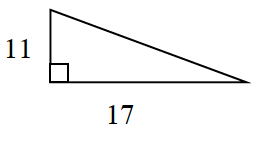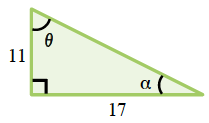### Home > INT2 > Chapter 8 > Lesson 8.1.1 > Problem8-16

8-16.Examine the triangle at right..

1. What is the perimeter of the triangle?

Use the Pythagorean Theorem.

2. What is the measure of each of the acute angles in the triangle?

You have learned many different trigonometry tools. Which is appropriate to the situation?

Label the acute angles, similar to those in the diagram below.$\tan(\theta) = \frac{17}{11}$

$\theta =\tan^{-1}\left (\frac{17}{11} \right ) \approx 57.1^\circ$

By the Triangle Angle Sum Theorem, $a$ must be
$\approx32.9^\circ$